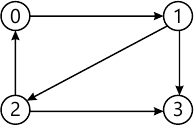MORE IN Data Structures and Applications
VTU Computer Science (Semester 3)
Data Structures and Applications
December 2013
Total marks: --
Total time: --
INSTRUCTIONS
(1) Assume appropriate data and state your reasons
(2) Marks are given to the right of every question
(3) Draw neat diagrams wherever necessary

1 (a) What is an ADT? Briefly explain the categories that classify the functions of a data type. Write an ADT for natural number.
10 M
1 (b) What is time complexity? Determine the time complexity of an iterative and recursive functions that adds n elements of the array using tabular method.
10 M

2 (a) Develop a structure to represent planets in the solar system. Each planet has fields for the planets name, its distance from the sum in miles and the number of moons it has. Write a program to read the data for each planet and store. Also print the name of the planet that has less distance from the sun.
8 M
2 (b) What is a polynomial? What is the degree of the polynomial? Write a function to add two polynomials?
8 M
2 (c) For the given sparse matrix A and its transpose, give the triplet representation. A is the given sparse matrix and B will be its transpose.
[ a=egin{bmatrix}15 &0 &0 &22 &0 &-15 \0 &11 &3 &0 &0 &0 \0 &0 &0 &-6 &0 &0 \0 &0 &0 &0 &0 &0 \91 &0 &0 &0 &0 &0 \0 &0 &-28 &0 &0 &0 end{bmatrix} ]
4 M

3 (a) Define stack. Implement push and pop functions for stack using arrays.
8 M
3 (b) Implement addq and deleteq functions for the circular queue.
6 M
3 (c) Write the postfix form of the following expressions
i) (a+b)*d+e/(f+a*b)+c
ii) ((a/(b-c+d))*(e-a)*c)
iii) a/b-c+d*e-a*c
6 M

4 (a) Write the different polynomial representation, with an example.
6 M
4 (b) For the given sparse matrix write the diagrammatic linked list representation?
[ A=egin{bmatrix} 5&0 &0 &0 \3 &0 &0 &1 \0 &0 &0 &0 \ 8&0 &0 &2 \0 &0 &7 &0 end{bmatrix} ]
6 M
4 (c) Define equivalence class. Write the linked list representation for the twelve polygons numbered 0 through 11 using the following pairs overlap?
0=4, 3=1, 6=10, 8=9, 7=4, 6=8, 3=5, 2=11, 11=0
8 M

5 (a) What is a tree? Explain:
i) Root node
ii) Degree
iii) Sibilings
iv) Depth of a tree and give examples.
8 M
5 (b) What is a binary tree? State its properties? How it is represented using array and linked list, given example?
8 M
5 (c) Define a max heap? Write a C function to insert an item into max heap?
4 M

6 (a) Explain the following, with an example
i) Selection trees
ii) Forests and its traversals.
8 M
6 (b) Describe the binary search tree with an example. Write a recursive function to search for a key value in a binary search tree.
8 M
6 (c) Write the adjacency matrix and adjacency list of the following graph.4 M

7 (a) Briefly explain the following, with an example:
i) HBLT ii) WBLT
8 M
7 (b) Write short notes on:
i) Priority queues
ii) Binomial heaps
iii) Fibonacci heaps.
12 M

8 (a) What is an AVL tree? Write the algorithm to insert an item into AVL tree.
8 M
8 (b) Explain the Red-Black tree? Briefly explain the different types of splay trees.
4 M

More question papers from Data Structures and Applications